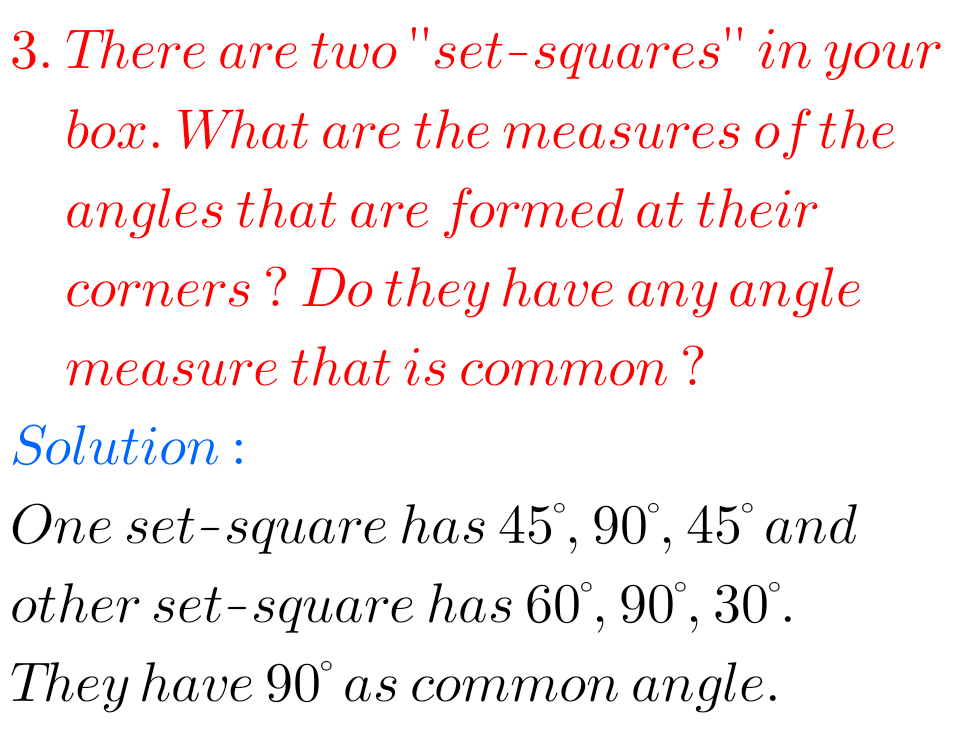## Exercise 5.5 chapter 5 class 6 Maths Ncert Solutions

Maths class 6 Understanding Elementary Shapes Solutions exercise 5.5 chapter 5 Ncert Maths Class 6 chapter 5 Understanding Elementary Shapes Exercise 5.5 solutions are given. Study the textbook lesson Understanding Elementary Shapes very well. Observe the example problems and solutions given in the textbook. Observe the solutons and try them in your own method. You …